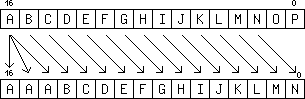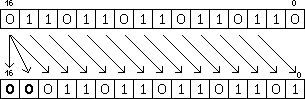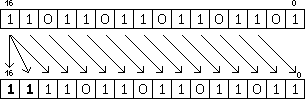version 2011-09-20

## SAR : Shift Arithmetic Right (divide signed by a power of two)

The bits of the source register (snd) are shifted towards the LSB, by a number of bits indicated by either

The MSBs of the result are filled with the original sign bit, while the logic shift (SHR) fills the MSB with 0s.

In the following examples, we will shift a register by two positions. This amounts to a division by 2^2=4. For YASEP16, the movement of the bits can be summarised by this diagram:For YASEP32, the principle is identical but with 32 bits instead of 16.

Let's consider that R1 gets loaded with 0110110110110110b, or 28086 in decimal. The unsigned shifting operation can be written this way:```mov 0110110110110110b r1
shr 2 r1```
28086/4=7021, which is equal to the binary result 0001101101101101b.

Unlike SHR, this instruction preserves the sign bit when we work with signed integers. For example with the value -9363, written 1101101101101101b in binary, we get this result:-9363/4=-2340, that is almost 1111011011011011=-2341
Indeed, right signed shifts round up for the negative numbers, but SAR is useful for more than these kinds of divisions.